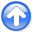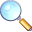Regularization is model stylingNext: Abandoned theory for matching Up: Regularization is model styling Previous: SEARCHING THE SEA OF

# CODE FOR THE REGULARIZED SOLVER

In Chapterwe defined linear interpolation as the extraction of values from between mesh points. In a typical setup (occasionally the role of data and model are swapped), a model is given on a uniform mesh, and we solve the easy problem of extracting values between the mesh points with subroutine lint1(). The genuine problem is the inverse problem, which we attack here. Data values are sprinkled all around, and we wish to find a function on a uniform mesh from which we can extract that data by linear interpolation. The adjoint operator for subroutine lint1() simply piles data back into its proper location in model space without regard to how many data values land in each region. Thus, some locations may receive much data while other locations get none. We could interpolate by minimizing the energy in the model gradient, in the second derivative of the model, or in any roughening model.

Formalizing now our wish that databe extractable by linear interpolation, from a model, and our wish that application of a roughening filter with an operatorhave minimum energy, we write the fitting goals:(14)

Suppose we take the roughening filter to be the second difference operatorscaled by a constant, and suppose we have a data point near each end of the model and a third data point exactly in the middle. Then, for a model space 6 points long, the fitting goal could look like:(15)

The residual vector has two parts, a data parton top and a model parton the bottom. The data residual should vanish except where contradictory data values happen to lie in the same place. The model residual is the roughened model.

Finding something unexpected is good science and engineering. We should look both in data space and model space. In data space, we look at the residual. In model space, we look at the residual projected there. After iterating to completion, we have, a sum of two images identical but for polarity. This image tells us what we have learned from the data; and how the model differs from what we thought it would be.

After all the definitions, we load the negative of the data into the residual. If a starting modelis present, then we update the data part of the residual, and we load the model part of the residual. Otherwise, we begin from a zero model; and thus, the model part of the residualis also zero. After this initialization, subroutine solver_reg() begins an iteration loop by first computing the proposed model perturbation(called g in the program) with the adjoint operator:(16)

Using this value of, we can find the implied change in residualas:(17)

and the last thing in the loop is to use the optimization step function stepper() to decide the length of the step size and to decide how much of the previous step to include.

An example of using the new solver is subroutine invint1. I chose to implement the model roughening operatorwith the convolution subroutine tcai1() which has transient end effects (and an output length equal to the input length plus the filter length). The adjoint of subroutine tcai1() suggests perturbations in the convolution input (not the filter).

user/gee/invint1.c
 void invint1(int niter /* number of iterations */, int nd /* data size */, float *coord /* data coordinates */, const float *dd /* data values */, int n1, float o1, float d1 /* model grid */, int na, const float *aa /* filter */, float *mm /* estimated model */, float eps /* regularization */) /*< inverse interpolation >*/ { lint1_init( o1, d1, coord); /* interpolation */ tcai1_init( na, aa); /* filtering */ sf_solver_reg(lint1_lop, sf_cgstep, tcai1_lop, n1+na, n1, nd, mm, dd, niter, eps); sf_cgstep_close( ); } 

Figure 11 shows an example for afilter with. The continuous curve representing the modelpasses through the data points. Because the models are computed with transient convolution end-effects, the models tend to damp linearly to zero outside the region where signal samples are given.

im1-2+1
Figure 11.
Sample points and estimation of a continuous function through them.To show an example where the result is clearly a theoretical answer, I prepared another figure with the simpler filter. When we minimize energy in the first derivative of the waveform, the residual becomes distributed uniformly between data points so the solution there is a straight line. Theoretically, it should be a straight line, because a straight line has a vanishing second derivative; and that condition arises by differentiating by, the minimized quadratic form, and getting. (By this logic, the curves between data points in Figure 11 must be cubics.) Theresult is shown in Figure 12.

im1-1a
Figure 12.
The same data samples and a function through them that minimizes the energy in the first derivative.The example of Figure 12 has been a useful test case for me. You will see it again in later chapters. What I would like to show you here is a movie showing the convergence to Figure 12. Convergence occurs rapidly where data points are close together. The large gaps, however, fill at a rate of one point per iteration.

SubsectionsRegularization is model stylingNext: Abandoned theory for matching Up: Regularization is model styling Previous: SEARCHING THE SEA OF

2014-12-03